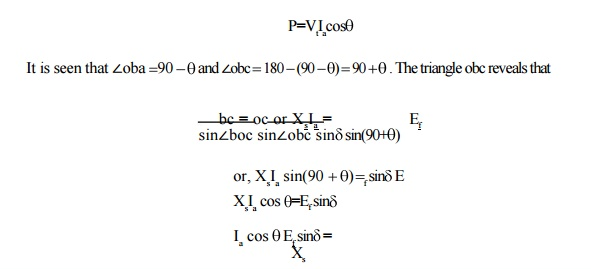Home | | Electrical Machines II | Power angle characteristics

# Power angle characteristics

When the synchronous generator feeding power to the infinite bus-bar at constant terminal voltage Vt as shown in single line diagram.

Power angle characteristics

When the synchronous generator feeding power to the infinite bus-bar at constant terminal voltage Vt as shown in single line diagram in Fig: 1.22 the phasor diagram for lagging power factor is shown if Fig: 1.23. For large size of generator armature resistance ra is negligible.The per phase power delivered to the infinite bus is given bySubstitution of value of Ia cosqin power equationThe variation of power as derived above with respect to power-angle is plotted in Fig; 1.24. The power versus load angle characteristic curve has a sinusoidal shape and is usually called power-angle characteristic of the cylindrical-rotor synchronous machine. The power P, for generator is taken as positive and therefore, for motor as negative.Study Material, Lecturing Notes, Assignment, Reference, Wiki description explanation, brief detail
Electrical machines : Synchronous Generator : Power angle characteristics |